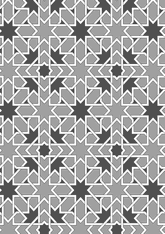data173/MOR2409

## Geometry

• The symmetry group of the tiling is *442 (p4m).
• All the internal angles of the constituent polygons are a multiple of 45°.
• Contains one regular 8-pointed star polygon with vertex angle of 90°.
• Contains two regular 8-pointed star polygons with vertex angle of 45°.
• There are 7 non-regular reflective tiles (including one dart).
• The tiling satisfies the interlace condition and has four finite interlaces and no infinite interlace with straight cross-overs.
• The tiling is edge-to-edge.
• As drawn, contains about 496 polygons.

## References

Publications referenced: